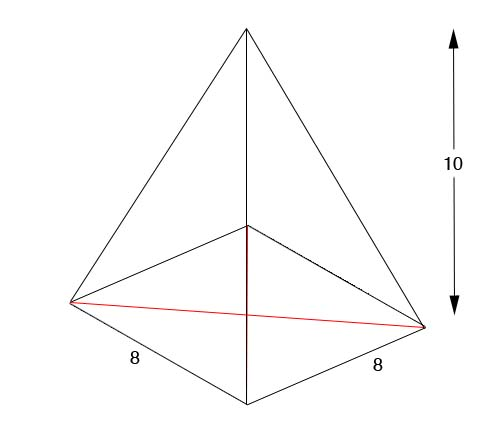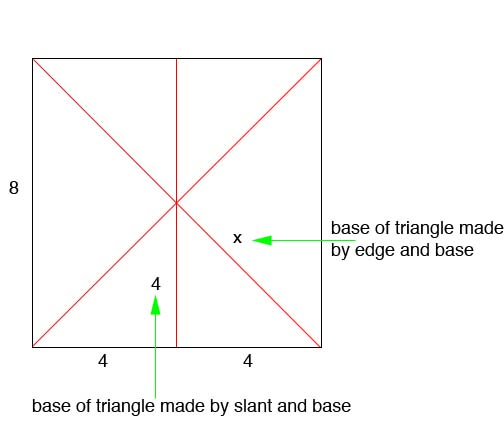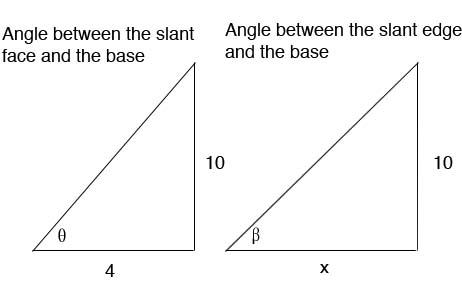SEARCH HOMEMath Central Quandaries & QueriesCarla, a student: A pyramid has its vertex directly above the centre of its square base. The edges of the base are each 8cm, and the vertical height is 10cm. Find the angle between the slant face and the base, and the angle between the slant edge and the baseHi Carla,

The key to finding the angles is making a triangle with the height of the pyramid.Looking at the base of pyramid, we can find the two bases of our triangles:What is x?

Next we can look at each of the triangles:Now that you know what x is, find your angles θ and β.

Hope this helps,

JaniceMath Central is supported by the University of Regina and The Pacific Institute for the Mathematical Sciences.